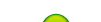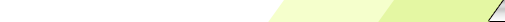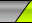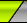Sábado, 2023-12-09My siteMain | Registration | LoginWelcome Visitante | RSS
 Statistics Total en línea: 1 Invitados: 1 Usuarios: 0

# Anticovidian v.2 COVID-19: Hypothesis of the Lab Origin Versus a Zoonotic Event which can also be of a Lab Origin: https://zenodo.org/record/3988139

To go to Einstein 5: http://fdocc.ucoz.com/index/0-90

# Einstein completes his observations:

As presented in the book "The Meaning of Relativity

"In 1921, five years after the appearance of his comprehensive paper on general relativity and twelve years before he left Europe permanently to join the Institute for Advanced Study, Albert Einstein visited Princeton University, where he delivered the Stafford Little Lectures for that year. These four lectures constituted an overview of his then controversial theory of relativity. Princeton University Press made the lectures available under the title The Meaning of Relativity, the first book by Einstein to be produced by an American publisher”.

"The General Theory of Relativity (Continued)” [Finiteness of the Universe]

… [I] shall give a brief discussion of the so-called cosmological problem… our previous considerations, based upon the field equations (96), had for a foundation the conception that space on the whole is Galilean-Euclidean, and that this character is disturbed only by asses embedded in it. This conception was certainly justified as long as we were dealing with spaces of the order of magnitude of those that astronomy has to do with. But whether portions of the universe, however large they may be, are quasi-Euclidean, is a wholly different question. We can make this clear by using an example from the theory of surfaces which we have employed many times. If a portion of a surface is observed by the eye to be practically plane, it does not at all follow that the whole surface has the form of a plane; the surface might just as well be a sphere, for example, of sufficiently large radius. The question as to whether the universe as a whole is non-Euclidean was much discussed from the geometrical point of view before the development of the theory of relativity. But with the theory of relativity, this problem has entered upon a new stage, for according to this theory the geometrical properties of bodies are not independent, but depend upon the distribution of masses [the field equation is represented as (96): Rmn  – ½gmn R = -kTmn ]…

…The possibility seems to be particularly satisfying that the universe is spatially bounded and… is of constant curvature, being either spherical or elliptical; for then the boundary conditions at infinity which are so inconvenient from the standpoint of the general theory of relativity, may be replaced by the much more natural conditions for a closed surface

Thus we may present the following arguments against the conception of a space-infinite, and for the conception of a space-bounded, universe:

1.     From the standpoint of the theory of relativity, the condition for a closed surface is very much simpler than the corresponding boundary condition at infinity of the quasi-Euclidean structure of the universe.

2.     The idea that Mach expressed, that inertia depends upon the mutual action of bodies, is contained, to a first approximation, in the equations of the theory of relativity; it follows from these equations that inertia depends, at least in part, upon mutual actions between masses. As it is an unsatisfactory assumption to make that inertia depends in part upon mutual actions, and in part upon an independent property of space, Mach’s idea gains in probability. But this idea of Match’s corresponds only to a finite universe, bounded in space, and not to a quasi-Euclidean, infinite universe. From the standpoint of epistemology it is more satisfying to have the mechanical properties of space completely determined by matter, and this is the case only in a space-bounded universe.

3.     An infinite universe is possible only if the mean density of matter in the universe vanishes. Although such an assumption is logically possible, it is less probable than the assumption that there is a finite mean density of matter in the universe.

Appendix for the Second Edition.

On the "Cosmologic Problem”

…The mathematician Friedman found a way out of this dilemma (the introduction of l (a universal constant, the "cosmologic constant”) he showed that it is possible, according to the field equations, to have a finite density in the whole (three-dimensional) space, without enlarging these field equations ad hoc. Zeitschr. F. Phys. 10 (1922)). His result then found a surprising confirmation by Hubble’s discovery of the expansion of the stellar system (a red shift of the spectral lines which increases uniformly with distance. The existence of the red shift of the spectral lines by the (negative) gravitational potential of the place of origin. This demonstration was made possible by the discovery of so-called "dwarf stars” whose average density exceeds that of water by a factor of the order 104. For such a star (e.g. the faint companion of Sirius), whose mass and radius can be determined (the mass is derived from the reaction on Sirius by spectroscopic means, using the Newtonian laws; the radius is derived from the total lightness and from the intensity of radiation per unit area, which may be derived from the temperature of its radiation), this red shift was expected, by the theory, to be about 20 times as large as for the sun, and indeed it was demonstrated to be within the expected range). The following is essentially nothing but an exposition of Friedman’s idea:

FOUR-DIMENSIONAL SPACE

WHICH IS ISOTROPIC WITH RESPECT TO

THREE DIMENSIONS

…The surfaces of constant radius are then surfaces of constant (positive) curvature which are everywhere perpendicular to the (radial) geodesics… There exists a family of surfaces orthogonal to the geodesics. Each of these surfaces is a surface of constant curvature

CHOICE OF COORDINATES

(3c)   A = (1/1 + cr2); B = 4c

c > 0 (spherical space)

c < 0 (pseudospherical space)

c = 0 (Euclidean space)

…we can further get in the first case c = ¼, in the second case c = -¼

In the spherical case the "circumference” of the unit space (G = 1) is

∫ [dr/1+(r2/4)] = 2p

[ ∫ going from infinite () to infinite() ]

the "radius” of the unit space is 1. In all three cases the function G of time is a measure for the change with time of the distance of two points of matter (measured on a spatial section). In the spherical case, G is the radius of space at the time x4.

THE FIELD EQUATIONS

… Since G is in all cases a relative measure for the metric distance of two material particles as function of time, G’/G expresses Hubble’s expansion…

THE SPECIAL CASE OF VANISHING SPATIAL CURVATURE

…The relation between Hubble’s expansion… and the average density…, is comparable to some extent with experience, at least as far as the order of magnitude is concerned. The expansion is given as 432 km/sec for the distance of 106 parsec…

…Can the present difficulty, which arouse under the assumption of a practically negligible spatial curvature, be eliminated by the introduction of a suitable spatial curvature?

SUMMARY AND OTHER REMARKS

(1)    The introduction of the "cosmologic member” (l) into the equation of gravity, though possible from the point of view of relativity, is to be rejected from the point of view of logical economy. As Friedman was the first to show one can reconcile an everywhere finite density of matter with the original form of the equations of gravity if one admits the time variability of the metric distance of two mass points [If Hubble’s expansion had been discovered at the time of the conception of the "general theory of relativity”, the cosmologic member (l) would never have been introduced. It seems now so much less justified to introduce such a member into the field equations, since its introduction loses its sole original justification, - that of leading to a natural solution of the cosmologic problem]

(2)    The demand for spatial isotropy of the universe alone leads to Friedman’s form. It is therefore undoubtedly the general form, which fits the cosmologic problem.

(3)    Neglecting the influence of spatial curvature, one obtains a relation between the mean density and Hubble’s expansion which, as to order of magnitude, is confirmed empirically...

(6) …It seems to me… that the "theory of evolution” of the stars rests on weaker foundations than the field equations.

…The "beginning of the world” (the "beginning of the expansion”) really constitutes a beginning, from the point of view of the development of the now existing stars and systems of stars, at which those stars and systems of stars did not yet exist as individual entities.

(8) For the reasons given it seems that we have to take the idea of an expanding universe seriously, in spite of the short "lifetime” (109). If one does so, the main question becomes whether space has positive (spherical case) or negative (pseudospherical case) spatial curvature

It is imaginable that the proof would be given that the world is spherical (it is hardly imaginable that one could prove it to be pseudospherical)…

Otros Trabajos de Einstein:

The World As I See It, an Essay by Albert Einstein

Relativity. The Special and General Theory

Compiló: Fernando Castro Chávez, Ph.D. Biología Molecular.

 Search
 Site friends Create your own site